# Project B3 • Frequency combs

### Principal investigators

 Prof. Dr. Tobias Jahnke (7/2015 - ) Prof. Dr. Christian Koos (7/2015 - ) Prof. Dr. Wolfgang Reichel (7/2015 - )

### Project summary

A frequency comb is an optical signal consisting of a multitude of equidistantly spaced optical frequencies. Since their discovery by Theodor Hänsch awarded with the Nobel prize in 2005, their tremendous applicability was demonstrated in e.g. optical metrology, frequency metrology and optical communications. In this project, we aim to find a mathematical and electrotechnical framework for the generation of frequency combs with several key characteristics.

Particularly interesting comb sources are nonlinear Kerr microresonators, cf. Figure 1. These chip-scale devices allow for the generation of frequency combs in setups with a footprint comparable to a matchbox, enabling their use in small and mobile objects such as autonomous cars, servers in data-centres and handhelds. In proof-of-principle experiments, we demonstrated the application of microresonator frequency combs in optical communications at data transmission rates of more than 50 Tbits/s through a single optical fiber, [Mea16, MPea17]. Furthermore, we performed optical distance measurements and achieved a sub-µm resolution at record sampling rates of 100 MHz [Gea17, Tea18].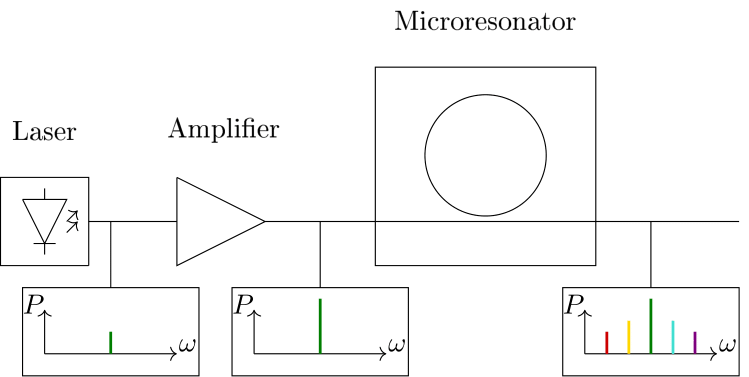Figure 1. Schematic of system for frequency comb generation. A tunable continuous wave (cw) laser emits light at a frequency $\omega$, which is amplified by an optical amplifier. Then, the light is coupled into a microresonator on a microchip. By tuning the frequency of the laser, a frequency comb emerges in the microresonator, which can then be coupled out of the chip again for further use.

To enable this technology for a widespread use, key characteristics such as energy efficiency and potential for mass production need to be investigated. We address these challenges in an interdisciplinary team consisting of mathematicians and engineers by phrasing practical demands and answering these with mathematical techniques. We focus on the optical power conversion efficiency of a driving optical field inside the resonator and the emerging frequency comb. Furthermore, we explore the potential of different material platforms. Here, especially Silicon is of interest due to its widespread use in the semiconductor industry.

From the mathematical point of view, the field inside the resonator is approximated by a complex-valued function $a(t,x)$, which depends on time $t$ and intracavity position $x$, and satisfies the Lugiato–Lefever–equation (LLE) $\frac{\partial a(t,x)}{\partial t}=\left[-1-\text{i}\zeta+\text{i}d\frac{\partial^2}{\partial x^2}+\text{i}|a(t,x)|^2\right]a(t,x)+f, \quad a(t,x+2\pi)=a(t,x)$ The LLE is a damped and forced nonlinear Schrödinger equation with parameters given by forcing $f$ (amplitude of the driving field), detuning $\zeta$ (relative offset between frequency of the driving field and a resonance frequency of the microresonator) and second-order dispersion $d$. When pumping the microresonator with an incoming single-frequency field, the Kerr-nonlinear term in the LLE gives rise to new spectral components, resulting in a frequency comb. Of most interest are soliton frequency comb states. These are stationary strongly localized solutions in space, which feature a broad spectrum in the frequency domain, cf. Figure 2.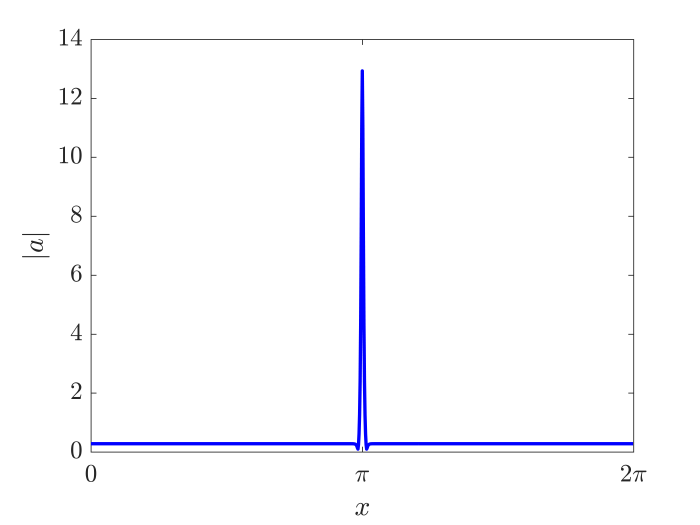Figure 2. Soliton solution and corresponding frequency comb for $d=0.01,\,\zeta=80$ and $f=23$.

#### New dynamical models for frequency combs in silicon-based microresonator

From the practical point of view, Kerr comb generation in silicon based microresonator is promising due to the high nonlinear Kerr effect of silicon and silicon is a well established material for photonic integrated circuits. However, the effects of two photon absorption (TPA) and free carrier absorption (FCA) work against comb formations. To investigate the possibility of comb formations, we formulated a modified variant of the LLE model taking into account TPA and FCA in silicon based microresonator. In [GMR22], existence of frequency combs in presence of TPA was shown, in [Tro20], the modulation instability occurs for certain TPA, FCA, and pump parameters.

TPA leads to a nonlinear damping, represented by the parameter $r > 0$. Additionally, free carriers generated by TPA will impact the refractive index via the free carrier dispersion parameter $\mu$. The density $N$ of the free carriers is governed by a first order ODE coupled to the modified LLE. The resulting dynamical models are described as: \begin{align*} \mathrm{i}\frac{\partial a(t,x)}{\partial t} &= \left[-\mathrm{i}+ \zeta - \sigma \left(\mathrm{i}- \mu \right)N(x-t\nu, t)-d \frac{\partial^2}{\partial x^2}-(1+\mathrm{i}r)|a(t,x)|^2 \right] a(t,x) + \mathrm{i}f,\\ \frac{\partial N(t,x)}{\partial t} &= r|a(x+t\nu, t)|^4-\frac{N(t,x)}{\tau}, \end{align*} where the positive quantities $\sigma$ and $\tau$ representing FCA cross-section and effective FCA lifetime. Since the field amplitude $a$ inside the microresonator is described in a reference frame moving with group velocity $\nu$ while the free carriers do not move, the offset ±$t\nu$ between the two reference frames is visible in the coupling of the two differential equations. In our investigations, the main findings are:

• Modulation instability occurs provided $r$ and $\sigma\tau$ stay below a critical threshold and simultaneously the pump power exceeds a critical value for sideband excitation, see Figure 3(a)
• Modulation instability is necessary for soliton comb formation - but not sufficient. Thus, dynamically increased detuning will drive the resonator into a final single soliton comb.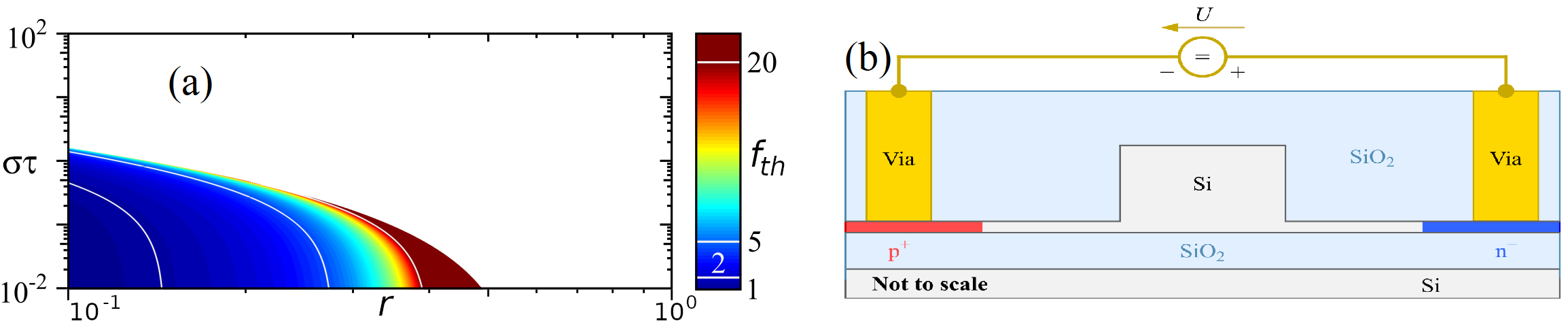Figure 3. (a) Critical $\sigma\tau$-threshold for modulation instability with color coded threshold power (anomalous dispersion). (b) Cross-section of the silicon rib waveguide surrounded by n-doped and p-doped regions, which forms a p-i-n junction. If the p-i-n junction is reverse biased, the two-photon generated free carriers will be removed.

Based on these results, we have started working towards an experimental demonstration of Kerr comb generation in silicon based microresonators, that are equipped with a reverse-biased p-i-n junction to sweep out two-photon generated free carriers from the waveguide core, see Figure~\ref{silicon_microresonator}(b).

#### Generation of frequency combs by two-mode pumping

In applications, generation of frequency combs by pumping two (instead of one) resonant modes of the ring resonator can be advantageous with respect to quality measures such as optical power conversion efficiency and bandwidth. In [GJKR22], [GPKR22] we investigate this novel pumping scheme modeled by the extended LLE: $$\frac{\partial u(t,x)}{\partial t} = \left[-1- \mathrm{i}\zeta + \mathrm{i}d \frac{\partial^2}{\partial x^2} + \mathrm{i} |u(t,x)|^2 \right] u(t,x) + f_0 + f_1 \mathrm{e}^{ \mathrm{i}(k_1 x - \nu_1 t)}.$$ Here the parameters $f_0, f_1$ model the power of the two lasers sources with total power $f_0^2+f_1^2 = f^2$, $k_1$ is the index of the second pumped mode and $\nu_1$ represents the frequency difference between the second laser source and the corresponding resonant mode $k_1$.

An obstacle for designing chip-scale frequency combs is that generation of frequency combs in a passive Kerr microresonator requires a continuous-wave laser as a pump, which is usually implemented by a bulky external cavity diode laser (ECDL). In [Mea22] we demonstrated comb generation using a chip-scale hybrid integrated external cavity laser (ECL). In fact, our devices generate Kerr-combs with intrinsic linewidth comparable to benchtop ECDL based combs, and thus we were able to show that Kerr frequency comb generators with narrow comb linewidth and compact footprint can be attained.

Using analytical and numerical continuation and bifurcation methods, cf. Fig. 4, we were able to prove the existence of traveling wave solutions to the extended LLE.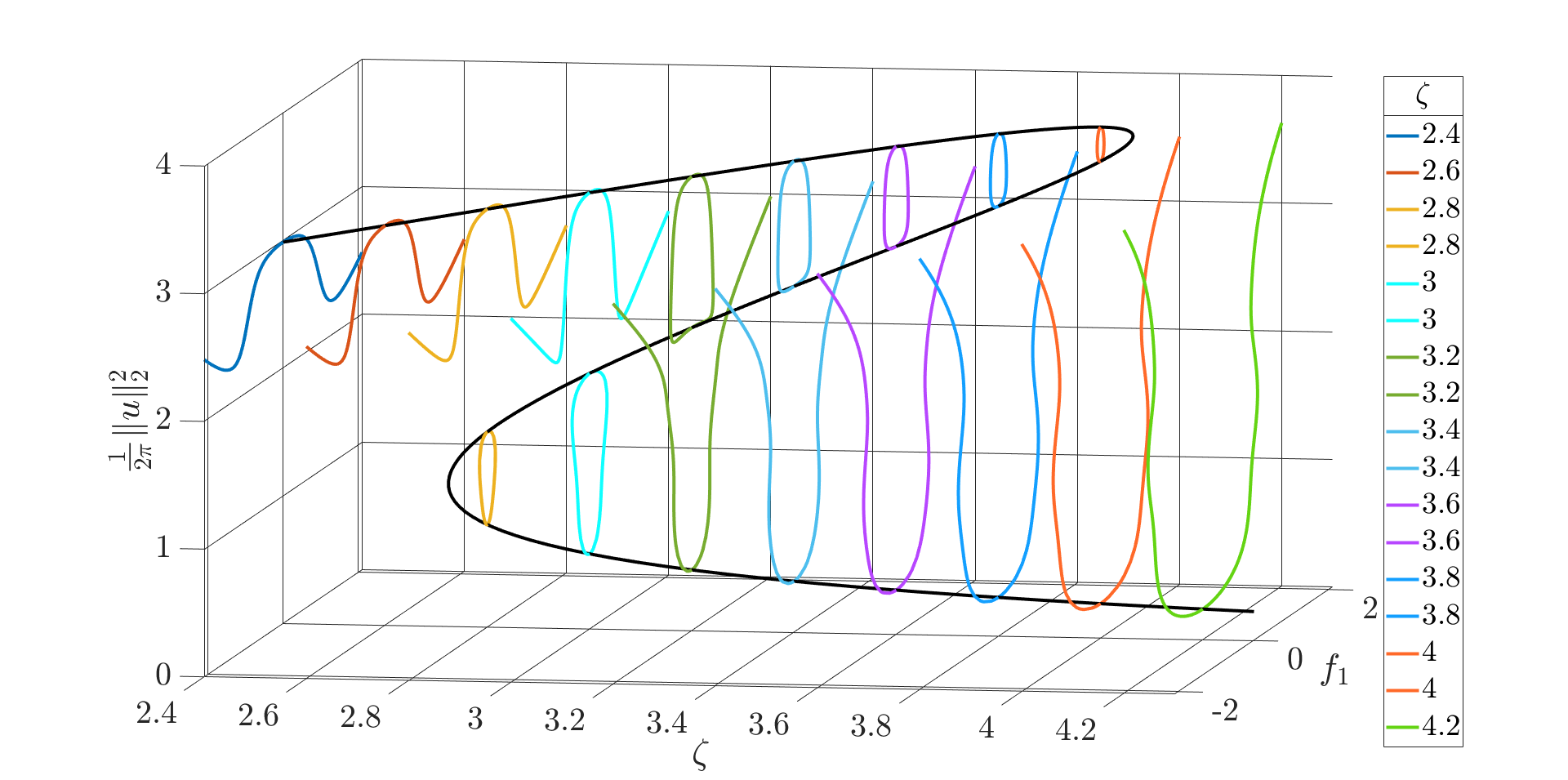Figure 4. Intracavity power $\|u\|_2^2$ of solutions of the extended LLE as a function of $\zeta$ and $f_1$. Colored lines correspond to non-trivial traveling wave solutions bifurcating from the constant solution curve (black line) for different values of $\zeta$.

Moreover, we numerically discovered that a symmetrical power distribution between the two lasers ($50 \%$ in each mode) simultaneously optimizes several important performance metrics of the Kerr-comb, see Fig. 5.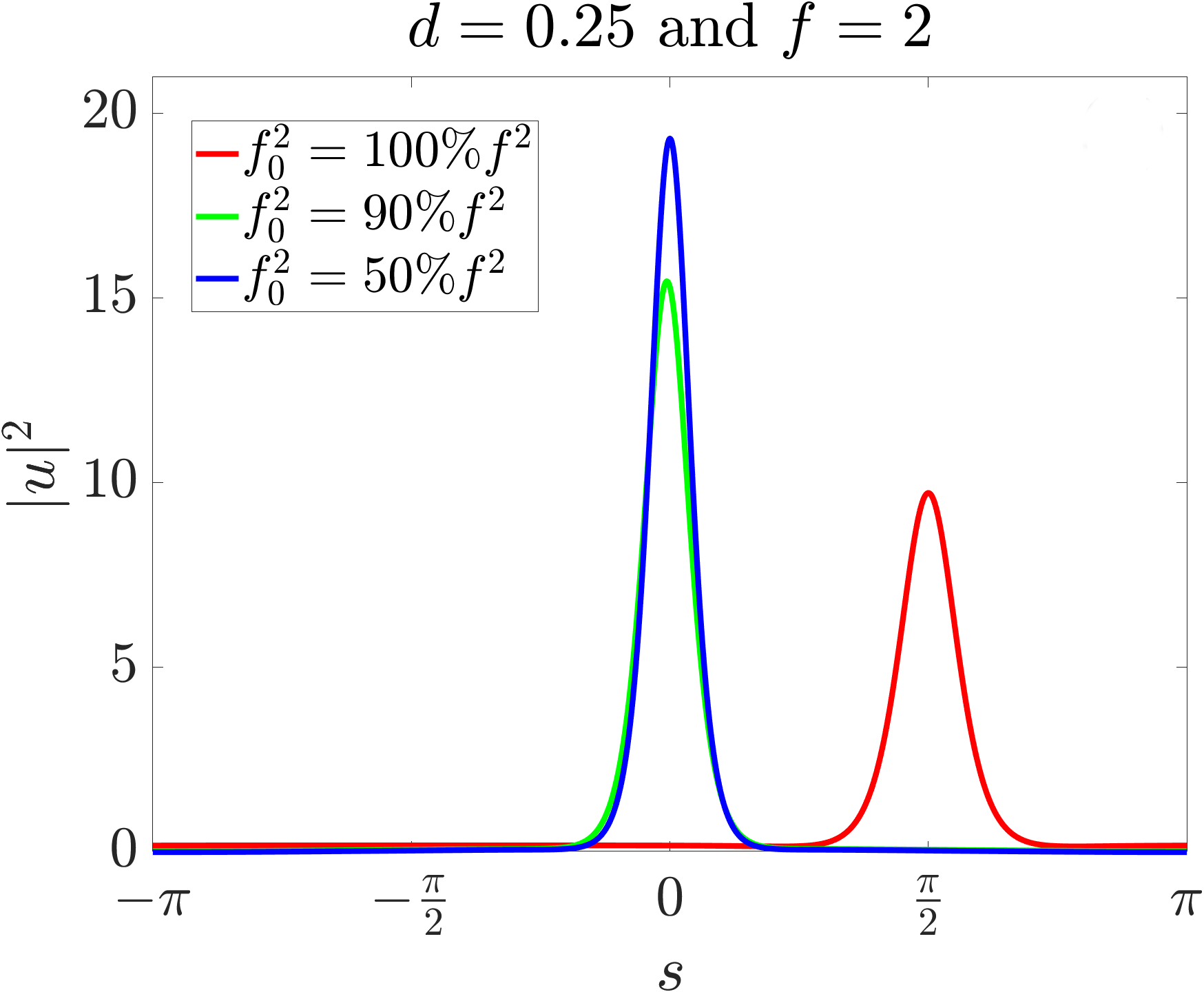Figure 5. Spatial and spectral power distributions of optimal solitons for total power $f = 2$ and distributions $f_0^2 = 100 \% f^2$, $f_0^2 = 90 \% f^2$ as well as $f_0^2 = 50 \% f^2$. The 50-50 case (blue case) yields the most localized solution with the broadest spectrum.

#### Applications of Kerr frequency combs

Apart from theoretical investigations and fundamental explorations of Kerr comb dynamics, we further demonstrated the viability of Kerr frequency combs in different application fields. In [MPea17], [MPea20], Kerr soliton combs were studied in the context of optical communications and experiments demonstrated their use for high-speed data transmission. Further applications include optical ranging [Tea18], and ultra-broadband photonic-electronic signal processing [Fea21], [Dea22]. Here, one of our main findings was that Kerr combs enable ultra-broadband analog-to-digital conversion with record-high acquisition bandwidth (320GHz) and optical waveform measurement with a record-high bandwidth exceeding 600GHz [Fea21], [Fea22], [Dea22].

An obstacle for designing chip-scale frequency combs is that generation of frequency combs in a passive Kerr microresonator requires a continuous-wave laser as a pump, which is usually implemented by a bulky external cavity diode laser (ECDL). In [Mea22] we demonstrated comb generation using a chip-scale hybrid integrated external cavity laser (ECL). In fact, our devices generate Kerr-combs with intrinsic linewidth comparable to benchtop ECDL based combs, and thus we were able to show that Kerr frequency comb generators with narrow comb linewidth and compact footprint can be attained.

#### Continuation and bifurcation for the Lugiato-Lefever equation

In order to find soliton states analytically, several approaches for the stationary equation $$-da'' + (\zeta - \mathrm{i}) a - |a|^2 u + \mathrm{i} f = 0$$ were used [MR17], [GR20]. Further, in [Gea19] we developed a heuristic to find the most localized solitons by numerical path continuation.

The first approach is continuation for $d>0$. We consider a reformulated version of the equation $-du''+(\zeta-\epsilon\text{i})u-|u|^2u+\text{i}f=0$ on the real line. For $\epsilon=f=0$ the soliton solution can be calculated explicitly, and then analytically continued into the regime where $\epsilon>0$ and $f>0$. This approach can also be used numerically as these solutions serve as approximations on a bounded interval. Scaling them back leads to a map of parameters where we can expect soliton solutions.

The second approach addresses bifurcation with respect to the parameter $\zeta$, cf. [MR17], and preprint [Mea16]. At certain values, non-trivial solutions bifurcate from the trivial curve, cf. Figure 6.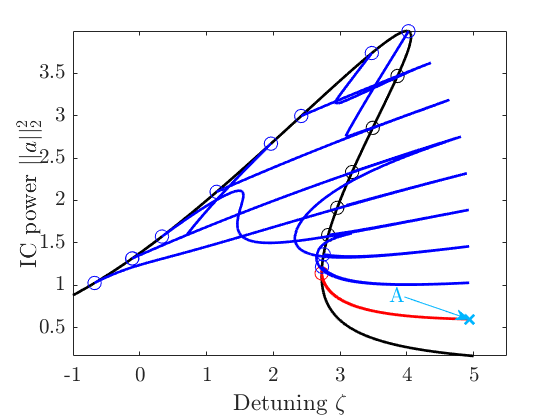Figure 6. Bifurcation diagrams for $d=0.1$ and $f=2$ (left) and for $d=-0.1$ and $f=2$ (right).

In our investigations, we observe the following heuristic:
For $d>0$ the most localized solitons are at the first turning point of the last bifurcating branch (Label A).
For $d<0$ the most localized solitons are at the second turning point of the first bifurcating branch (Label B).
Using this heuristic, we can identify parameter regimes where the most localized soliton solutions are found. Defining quality measures such as combwidth and power conversion efficiency, this enables us to determine a universal conversion efficiency map, cf. Figure 4, which is essential for designing microresonators embedded in integrated photonic systems, cf. [Gea19].Figure 4. Combwidth and power conversion efficiency for $d>0$ and $d<0$.

Within our project we also consider numerical time integration of the LLE via a Strang-Splitting between its nonlinear and dispersive part; cf. [JMS17]. One of our observation was that time integration follow the bifurcation branches when the detuning is swept over time.

## Publications

1. , , and . The Lugiato–Lefever equation with nonlinear damping caused by two photon absorption. J. Dyn. Diff. Equat., 34(3):2201–2227, September . [preprint] [bibtex]

2. and . Soliton solutions for the Lugiato–Lefever equation by analytical and numerical continuation methods. In W. Dörfler, M. Hochbruck, D. Hundertmark, W. Reichel, A. Rieder, R. Schnaubelt, and B. Schörkhuber, editors, Mathematics of Wave Phenomena, Trends in Mathematics, pages 179–195, October . Birkhäuser Basel. [bibtex]

3. , , , , , and . Performance of chip-scale optical frequency comb generators in coherent WDM communications. Opt. Express, 28(9):12897–12910, April . [bibtex]

4. , , , , , and . Bandwidth and conversion efficiency analysis of dissipative Kerr soliton frequency combs based on bifurcation theory. Phys. Rev. A, 100(3):033819, September . [preprint] [bibtex]

5. . Global secondary bifurcation, symmetry breaking and period-doubling. Topol. Methods Nonlinear Anal., 53(2):779–800, June . [preprint] [bibtex]

6. , , , , , , , , , , , , and . Ultrafast optical ranging using microresonator soliton frequency combs. Science, 359(6378):887–891, February . [bibtex]

7. , , and . Strang splitting for a semilinear Schrödinger equation with damping and forcing. J. Math. Anal. Appl., 455(2):1051–1071, November . [preprint] [bibtex]

8. , , , , , , , , , , , , , , and . Microresonator-based solitons for massively parallel coherent optical communications. Nature, 546:274–279, June . [bibtex]

9. , , , , , , , , , , , and . Ultrafast dual-comb distance metrology using dissipative Kerr solitons. In Conference on Lasers and Electro-Optics, STh4L.6, May . Optical Society of America. [bibtex]

10. and . A priori bounds and global bifurcation results for frequency combs modeled by the Lugiato-Lefever equation. SIAM J. Appl. Math., 77(1):315–345, February . [preprint] [bibtex]

11. , , , , , , , , , and . 34.6 Tbit/s WDM transmission using soliton Kerr frequency combs as optical source and local oscillator. In European Conference on Optical Communication (ECOC), pages 415–417, December . [bibtex]

12. , , , , , , , , , , , , , and . 50 Tbit/s massively parallel WDM transmission in C and L band using interleaved cavity-soliton Kerr combs. In Conference on Lasers and Electro-Optics, STu1G.1, June . [preprint] [bibtex]

## Preprints

1. , , , , , , , , , , , , and . Sub-kHz-linewidth external-cavity laser (ECL) with Si${}_3$N${}_4$ resonator used as a tunable pump for a Kerr frequency comb. Preprint, Karlsruhe Institute of Technology (KIT), November . [bibtex]

2. , , , and . Global continua of solutions to the Lugiato–Lefever model for frequency combs obtained by two-mode pumping. CRC 1173 Preprint 2022/56, Karlsruhe Institute of Technology, October . [bibtex]

3. , , , and . Bandwidth and conversion-efficiency analysis of Kerr soliton combs in dual-pumped resonators with anomalous dispersion. CRC 1173 Preprint 2022/55, Karlsruhe Institute of Technology, October . [bibtex]

4. and . Stochastic Galerkin-collocation splitting for PDEs with random parameters. CRC 1173 Preprint 2018/28, Karlsruhe Institute of Technology, October . [bibtex]

## Theses

1. . On the Lugiato–Lefever model for frequency combs in a dual-pumped ring resonator with an appendix on band structures for periodic fractional Schrödinger operators. PhD thesis, Karlsruhe Institute of Technology (KIT), November . [bibtex]

2. . Chip-scale optical frequency comb sources for terabit communications. PhD thesis, Karlsruhe Institute of Technology (KIT), February . [bibtex]

3. . Kerr-nonlinear microresonators and frequency combs: modelling, design and applications. PhD thesis, Karlsruhe Institute of Technology (KIT), November . [bibtex]

4. . Continuation and bifurcation of frequency combs modeled by the Lugiato–Lefever equation. PhD thesis, Karlsruhe Institute of Technology (KIT), February . [bibtex]

5. . Terabit-rate transmission using optical frequency comb sources. PhD thesis, Karlsruhe Institute of Technology (KIT), July . [bibtex]

## Other references

1. , , , , , , , , , , , , and . Slice-less optical arbitrary waveform measurement (OAWM) in a bandwidth of more than 600 GHz. In 2022 Optical Fiber Communications Conference and Exhibition (OFC), pages 1–3, March . [bibtex]

2. , , , , , , , , , , , , , , and . Optical arbitrary waveform measurement (OAWM) using silicon photonic slicing filters. J. Light. Technol., 40(6):1705–1717, March . [bibtex]

3. , , , , , , , , , , , , , , , , , , and . 320 GHz analog-to-digital converter exploiting Kerr soliton combs and photonic-electronic spectral stitching. In 2021 European Conference on Optical Communication (ECOC), pages 1–4, September . [bibtex]

Former staff
Name Title Function
Dipl.-Phys. Doctoral researcher
Dr. Doctoral researcher
Dr. Doctoral researcher
M.Sc. Doctoral researcher
M.Sc. Doctoral researcher
Dr.-Ing. Doctoral researcher
Dr. Doctoral Researcher